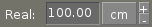<-- Back to AG_Intro.3

# SYNOPSIS

 ```#include #include ```

# DESCRIPTIONThe AG_Numerical widget allows the value of an integer or floating-point variable to be edited by the user. AG_Numerical provides a value binding, and optional limits may be specified as the min and max bindings. If min or max are undefined when the widget is first shown, default limits are set according to the widest numerical range that can be represented by the type of value. AG_Numerical is composed of an AG_Textbox(3), two AG_Button(3) widgets to increment and decrement the value, and optionally an AG_UCombo(3) for selecting units.

# INHERITANCE HIERARCHY

 AG_Object(3)-> AG_Widget(3)-> AG_Numerical.

# INITIALIZATION

 AG_Numerical * AG_NumericalNew (AG_Widget *parent, Uint flags, const char *unit, const char *format, ...)AG_Numerical * AG_NumericalNewS (AG_Widget *parent, Uint flags, const char *unit, const char *label) AG_Numerical * AG_NumericalNew{Flt,Dbl,Ldbl,Int,Uint} (AG_Widget *parent, Uint flags, const char *unit, const char *label, *value) AG_Numerical * AG_NumericalNew{Flt,Dbl,Ldbl,Int,Uint}R (AG_Widget *parent, Uint flags, const char *unit, const char *label, *value, min, max)void AG_NumericalSizeHint (AG_Numerical *num, const char *sizeHint)int AG_NumericalSetUnitSystem (AG_Numerical *num, const char *unit)void AG_NumericalSelectUnit (AG_Numerical *num, const char *unit)void AG_NumericalSetPrecision (AG_Numerical *num, const char *fmt, int precision)void AG_NumericalSetWriteable (AG_Numerical *num, int writeable)

The AG_NumericalNew() function allocates, initializes, and attaches a new AG_Numerical widget. Acceptable flags include:
 AG_NUMERICAL_INT If no "value" binding has been set when the widget is first shown, use a built-in variable of type int (by default, a built-in double is used). AG_NUMERICAL_EXCL No external changes to the value binding are to be expected and AG_Numerical can perform optimizations based on the assumption of exclusive access (by default, the value is checked periodically and the widget is redrawn if a change is detected). AG_NUMERICAL_SLOW In non-EXCL case, check value every 2 seconds instead of the default of 250ms. AG_NUMERICAL_HFILL Expand horizontally in parent container. AG_NUMERICAL_VFILL Expand vertically in parent container. AG_NUMERICAL_EXPAND Shorthand for AG_NUMERICAL_HFILL AG_NUMERICAL_VFILL|.

The unit argument is the name of the conversion unit that will be used by default (other conversion units in the same unit group will also be displayed in the unit selector). If unit is NULL, no conversion is done and the unit selector is not shown. See AG_Units(3) for more information.

The alternate constructor routines AG_NumericalNew<Type>() create a new widget bound to a variable of the specified type. AG_NumericalNew<Type>R() also accept explicit min and max arguments.

AG_NumericalSizeHint() requests that the initial geometry of the AG_Numerical widget be sufficient to display sizeHint in its entirety (see AG_SizeSpec(3)).

AG_NumericalSetUnitSystem() searches all unit groups for a unit of the given name and selects it as the active unit. Other units in the group will be displayed in the unit selector. If no such unit exists, the function returns -1.

AG_NumericalSelectUnit() searches only the current unit group and selects the unit of the given name as the active conversion unit.

The AG_NumericalSetPrecision() function selects the preferred style and precision for printing floating-point numbers. The default is %.2f.

AG_NumericalSetWriteable() enables of disables user modification sets the writeability flag, also accessible as the writeable member of the structure.

# NUMERICAL OPERATIONS

 void AG_NumericalIncrement (AG_Numerical *num)void AG_NumericalDecrement (AG_Numerical *num)float AG_NumericalGetFlt (AG_Numerical *num)double AG_NumericalGetDbl (AG_Numerical *num)

The AG_NumericalIncrement() and AG_NumericalDecrement() functions increment and decrement the bound value by the interval specified as the inc binding (which defaults to 1).

AG_NumericalGetFlt(), AG_NumericalGetDbl() and AG_NumericalGetLdbl() return a representation of the current numerical value.

# STRUCTURE DATA

For the AG_Numerical object:
 char *format Format string for printing floating-point numbers. const AG_Unit *unit Current unit system in use. AG_Textbox *input Textbox for keyboard input.

# EVENTS

The AG_Numerical widget generates the following events:
 numerical-changed (void) The value has been modified by the textbox or the +/- buttons. numerical-return (void) The value has been modified by the textbox and return was pressed.

# BINDINGS

The AG_Numerical widget provides the following bindings:
 float *value, *min, *max, *inc Real number (single-precision). double *value, *min, *max, *inc Real number (double-precision). int *value, *min, *max, *inc Signed integer value. Uint *value, *min, *max, *inc Unsigned integer value. Uint8 *value, *min, *max, *inc Unsigned 8-bit value. Uint16 *value, *min, *max, *inc Unsigned 16-bit value. Uint32 *value, *min, *max, *inc Unsigned 32-bit value. Uint64 *value, *min, *max, *inc Unsigned 64-bit value. Sint8 *value, *min, *max, *inc Signed 8-bit value. Sint16 *value, *min, *max, *inc Signed 16-bit value. Sint32 *value, *min, *max, *inc Signed 32-bit value. Sint64 *value, *min, *max, *inc Signed 64-bit value.

The value binding indicates the number to display. The min and max bindings allow a range to be specified. The inc binding specifies the increment effected by the widget's "+" and "-" buttons (if unit conversion is in use, inc should be of the same unit system as value).

The 64-bit types are only available if AG_HAVE_64BIT is defined.